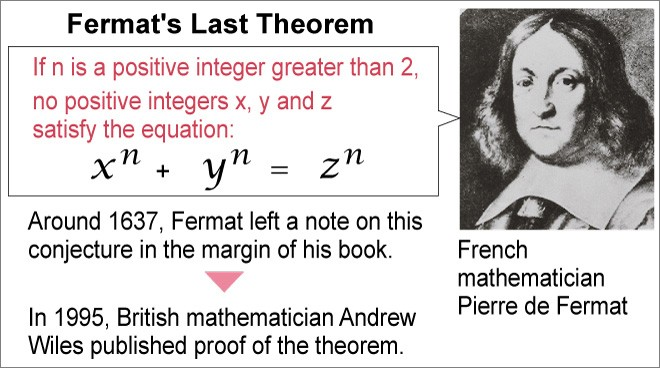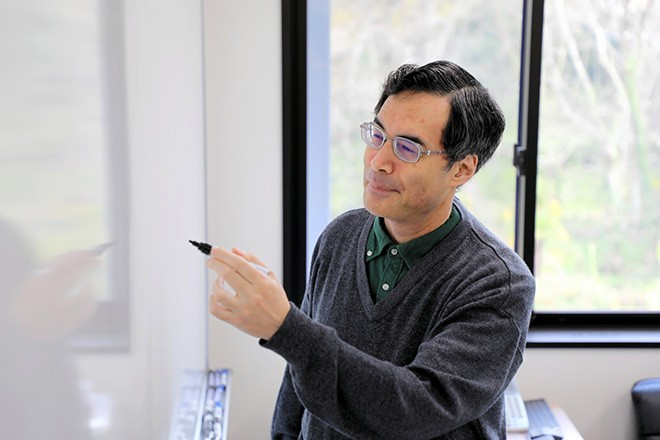The Asahi Shimbun

For more than 350 years, a mathematics problem whose solution was considered the Holy Grail to the greatest mathematician minds had remained unsolved.

Now, a team of mathematicians led by a prominent Kyoto University professor has offered an alternative proof to Fermat's Last Theorem, the potential second solution in the past quarter-century.

Shinichi Mochizuki, a professor at Kyoto University’s Research Institute for Mathematical Sciences (RIMS), spent about 20 years working solo on what he calls "Inter-universal Teichmuller (IUT) Theory."

The groundbreaking theory combines elements of algebra with geometry to create a link between a countless number of "universes" that contain tools for mathematics to enable addition and multiplication to freely travel between those universes.

Mochizuki used the theory to solve what has been called "ABC Conjecture," a brainteaser concerning a number theory conjecture, which had baffled the greatest minds since it was first proposed in 1985.

In an interview with The Asahi Shimbun, Mochizuki called using IUT Theory to provide a proof of Fermat's Last Theorem a "byproduct" of his team's work.

The editorial board of Kodai Mathematical Journal (Kodai Math.J.), published by the Tokyo Institute of Technology, said it accepted an academic paper on the study submitted by the team and that it is set to be published soon.

This spring, Kyoto University’s mathematical journal published Mochizuki's papers in which he outlined his solution to the ABC Conjecture.

Since the publication of the papers, mathematicians have had high hopes that IUT Theory could also be used to solve other math problems because the theory goes beyond the now accepted framework for mathematics.

TOOK MORE THAN 350 YEARS TO SOLVE

Fermat's Last Theorem is a conjecture stated around 1637 by the French mathematician Pierre de Fermat that if n is a positive integer greater than 2, no positive integers x, y and z satisfy the equation: x^n + y^n = z^n (x to the power of n + y to the power of n = z to the power of n).

If n is 2, the equation has a countless number of positive integral solutions for x, y and z. That proposition is known as Pythagorean Theorem.

But proving Fermat's Last Theorem has been a near impossibility over the centuries. It had remained unsolved until the British mathematician Andrew Wiles published proof of the theorem in 1995 by solving part of the so-called Taniyama-Shimura conjecture for elliptic curves.

For his proof of Fermat's Last Theorem, Wiles received a silver plaque as a special tribute from the International Mathematical Union, which awards the Fields Medal, considered the Nobel Prize for mathematicians, to recognize outstanding mathematical achievement.

Fermat scribbled a famous note on the theorem in the margin of one of the books in his library. In the note, he wrote that he has discovered a truly remarkable proof of the theorem, which the margin is too small to include.

The proposition became known as Fermat’s “Last” Theorem because it was the only one that had remained unsolved for more than 350 years among many notes he left in books in his library.

Mochizuki’s team comprises five mathematicians, including Yuichiro Hoshi, an associate professor at RIMS, and Ivan Fesenko, a professor at the University of Nottingham’s School of Mathematics.

Using an enhanced IUT Theory, they derived a “special inequality" concerning addition and multiplication and incorporated it into the equation of Fermat's Last Theorem to give an alternative proof to the theorem.

The team said identifying the value of a previously unknown variable in the inequality led to a breakthrough in proving the theorem. Last year, the team announced that it mostly proved the theorem, but it said its latest proof, a modified version of the previous one, is “complete.”Shinichi Mochizuki, a professor at Kyoto University’s Research Institute for Mathematical Sciences (Provided by Kyoto University)

IUT THEORY 'WONDERFUL TOOL FOR MATHEMATICIANS'

The ABC Conjecture and Fermat's Last Theorem are called Diophantine problems, where mathematicians try to determine whether certain equations have solutions. Many Diophantine problems are said to be tricky.

Fesenko said the team’s latest paper is the "second confirmed proof" of Fermat's Last Theorem because no one else has given a proof since Wiles did. Fesenko also said IUT Theory is a wonderful tool for mathematicians.

"The development of IUT Theory, like this one, should open the way to approaching various difficult (math) problems other than the ABC Conjecture," he said.

The concept of IUT Theory is so complex that it is believed that only around 10 people in the world can fully understand it. Opinions are divided among mathematicians over the evaluation of the theory.

A German mathematician has pointed out Mochizuki failed to provide a sufficient proof of the ABC Conjecture.

But the team’s presentation of a new application of IUT Theory may help encourage other mathematicians to learn the theory.

Mochizuki has shunned requests for media interviews about his work, but he responded to a request for an interview by The Asahi Shimbun for the first time.

“If that (other mathematicians start learning the theory) occurs, I will welcome it,” he said in a long email reply.

He wrote that he is currently working on a fundamental enhancement of IUT Theory.

The paper was posted on Nov. 8 on the website of Mochizuki’s laboratory: (https://www.kurims.kyoto-u.ac.jp/~motizuki/Explicit%20estimates%20in%20IUTeich.pdf).